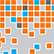# Online Program

Saturday, June 1New Developments in Statistical Learning
Sat, Jun 1, 2:45 PM - 3:50 PM
Regency Ballroom AB

### Quantile Regression for Big Data with Small Memory (306246)

Xi Chen, New York University
Weidong Liu, Shanghai Jiao Tong University
*Yichen Zhang, New York University

Keywords: distributed inference, divide-and-conquer, quantile regression, large scale data analysis, online streaming data

The development of modern technology has enabled data collection of unprecedented size, which poses new challenges to many statistical estimation and inference problems. We study the statistical inference problem in quantile regression (QR) for large-scale datasets that cannot be fit into memory or are distributed in many machines over limited memory. A natural method is the naive divide-and-conquer (DC) approach, which splits data into batches, computes the local QR estimator for each batch, and then aggregates the estimators via averaging. Despite its simplicity, the DC estimator solves a non-smooth optimization for each batch of the data, which is computationally expensive. Moreover, to achieve the same asymptotic efficiency as pooling all the data together, the DC estimator has a constraint that the number of batches cannot be too large, which limits its potential applications (e.g., in a large-scale sensor network).

To address the challenges, we propose a new linear-type estimator for QR (LEQR), and further developed a computationally efficient procedure that can successively refine the estimator via multiple rounds of aggregations. Theoretically, by establishing the Bahadur representation of the LEQR with an optimal rate reminder term, we established the asymptotic normality of the estimator, and further show that, without any constraint on the number of batches, our estimator achieves the same efficiency as the QR estimator computed on all the data. Moreover, our result allows the case that the dimensionality goes to infinity. Our proposed method is directly applied to the distributed setting. On each local machine, only matrix multiplication and summation need to be computed, and the communication cost is low. In addition, for online streaming data which arrives at the processor at a high speed, we develop a one-pass variant of our proposed estimator (online LEQR) that achieves the same statistical efficiency as the standard QR estimator.